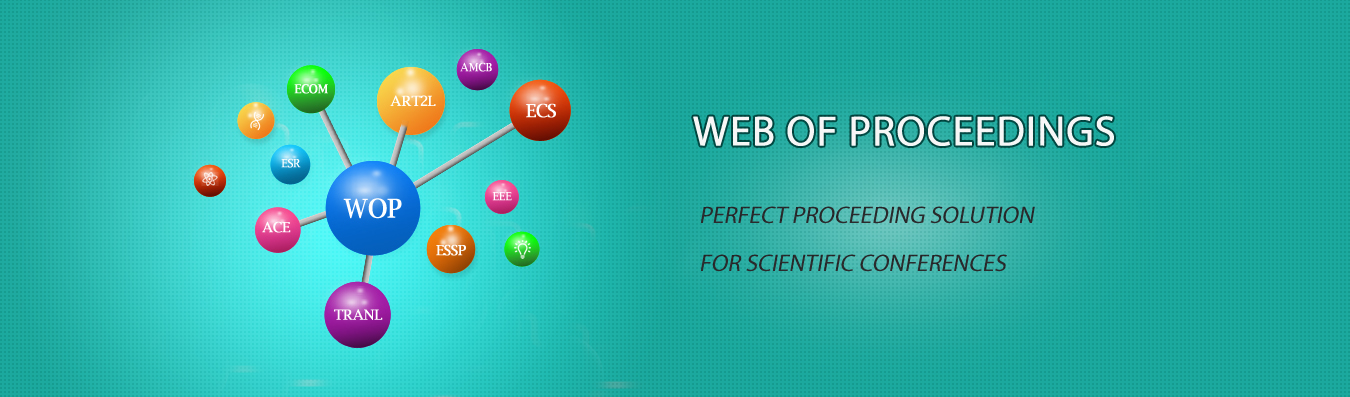The best way to conference proceedings by Francis Academic Press### Practical Research on the Integration of Mathematical Modeling Thought into Function Teaching

DOI: 10.25236/etmhs.2019.375

Jixia Deng

Jixia Deng

#### Abstract

Through the joint study of theory and practice, this paper uses the method of literature review to understand the general situation of the development of mathematical modeling in China and abroad, and then discusses the role, steps and methods of integrating the idea of mathematical modeling into function teaching. Mathematical modeling has played a great role in students'interest in learning mathematics, mathematics learning methods, mathematical problem solving ideas and comprehensive practical ability. At the same time, it also analyses various factors that affect the development of mathematical modeling in middle schools. It is found that the main factors hindering the development of mathematical modeling in middle schools come from four aspects: teachers, students, parents and society.

#### Keywords

Mathematical Modeling Thought, Function Teaching, Step-by-step Into, Effective